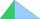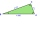# Right triangle calculator (A)

Please enter two properties of the right triangle

Use symbols: a, b, c, A, B, h, T, p, r, R

You have entered area T and angle α.

### Right scalene triangle.

Sides: a = 55.0000297247   b = 76.9999583856   c = 8.60223086813

Area: T = 17.5
Perimeter: p = 20.60222967917
Semiperimeter: s = 10.30111483958

Angle ∠ A = α = 35.538° = 35°32'17″ = 0.62202551096 rad
Angle ∠ B = β = 54.462° = 54°27'43″ = 0.95105412172 rad
Angle ∠ C = γ = 90° = 1.57107963268 rad

Height: ha = 76.9999583856
Height: hb = 55.0000297247
Height: hc = 4.06986752006

Median: ma = 7.43330001825
Median: mb = 6.10332902273
Median: mc = 4.30111543407

Vertex coordinates: A[8.60223086813; 0] B[0; 0] C[2.90662311264; 4.06986752006]
Centroid: CG[3.83661799359; 1.35662250669]
Coordinates of the circumscribed circle: U[4.30111543407; 0]
Coordinates of the inscribed circle: I[3.30111900102; 1.69988397145]

Exterior (or external, outer) angles of the triangle:
∠ A' = α' = 144.462° = 144°27'43″ = 0.62202551096 rad
∠ B' = β' = 125.538° = 125°32'17″ = 0.95105412172 rad
∠ C' = γ' = 90° = 1.57107963268 rad

# How did we calculate this triangle?

The calculation of the triangle progress in two phases. The first phase is such that we try to calculate all three sides of the triangle from the input parameters. The first phase is different for the different triangles query entered. The second phase is the calculation of other characteristics of the triangle, such as angles, area, perimeter, heights, the center of gravity, circle radii, etc. Some input data also results in two to three correct triangle solutions (e.g., if the specified triangle area and two sides - typically resulting in both acute and obtuse) triangle).

### 1. Input data entered: angle α and area T

$α = 35.538^\circ \ \\ T = 17.5$

### 2. From angle α we calculate angle β:

$α + β + 90^\circ = 180^\circ \ \\ β = 90^\circ - α = 90^\circ - 35.538 ^\circ = 54.462 ^\circ$

### 3. From area T, angle α and angle β we calculate hypotenuse c:

$c^2 \ \sin α \sin β = 2 \ T \ \\ c = \sqrt{ \dfrac{ 2 \ T }{ \sin α \sin β } } \ \\ c = \sqrt{ \dfrac{ 2 \cdot \ 17.5 }{ \sin 35^\circ 32'17" \cdot \ \sin 54^\circ 27'43" } } = 8.602$

### 4. From area T and hypotenuse c we calculate height h:

$T = \dfrac{ c \cdot \ h }{ 2 } \ \\ h = 2 \cdot \ T / c = 2 \cdot \ 17.5 / 8.602 = 4.069$

### 5. From hypotenuse c and angle α we calculate cathetus a:

$\sin α = a:c \ \\ a = c \cdot \ \sin α = 8.602 \cdot \ \sin(35.538 ^\circ ) = 5$

### 6. From cathetus a and hypotenuse c we calculate cathetus b - Pythagorean theorem:

$c^2 = a^2+b^2 \ \\ b = \sqrt{ c^2 - a^2 } = \sqrt{ 8.602^2 - 5^2 } = 7$

Now we know the lengths of all three sides of the triangle, and the triangle is uniquely determined. Next, we calculate another its characteristics - same procedure as calculation of the triangle from the known three sides SSS.

$a = 5 \ \\ b = 7 \ \\ c = 8.6$

### 7. The triangle perimeter is the sum of the lengths of its three sides

$p = a+b+c = 5+7+8.6 = 20.6$

### 8. Semiperimeter of the triangle

The semiperimeter of the triangle is half its perimeter. The semiperimeter frequently appears in formulas for triangles that it is given a separate name. By the triangle inequality, the longest side length of a triangle is less than the semiperimeter.

$s = \dfrac{ p }{ 2 } = \dfrac{ 20.6 }{ 2 } = 10.3$

### 9. The triangle area - from two legs

$T = \dfrac{ ab }{ 2 } = \dfrac{ 5 \cdot \ 7 }{ 2 } = 17.5$

### 10. Calculate the heights of the right triangle from its area.

$h _a = b = 7 \ \\ \ \\ h _b = a = 5 \ \\ \ \\ T = \dfrac{ c h _c }{ 2 } \ \\ \ \\ \ \\ h _c = \dfrac{ 2 \ T }{ c } = \dfrac{ 2 \cdot \ 17.5 }{ 8.6 } = 4.07$

### 11. Calculation of the inner angles of the triangle - basic use of sine function

$\sin α = \dfrac{ a }{ c } \ \\ α = \arcsin(\dfrac{ a }{ c } ) = \arcsin(\dfrac{ 5 }{ 8.6 } ) = 35^\circ 32'17" \ \\ \sin β = \dfrac{ b }{ c } \ \\ β = \arcsin(\dfrac{ b }{ c } ) = \arcsin(\dfrac{ 7 }{ 8.6 } ) = 54^\circ 27'43" \ \\ γ = 90^\circ$

An incircle of a triangle is a circle which is tangent to each side. An incircle center is called incenter and has a radius named inradius. All triangles have an incenter, and it always lies inside the triangle. The incenter is the intersection of the three angle bisectors. The product of the inradius and semiperimeter (half the perimeter) of a triangle is its area.

$T = rs \ \\ r = \dfrac{ T }{ s } = \dfrac{ 17.5 }{ 10.3 } = 1.7$

The circumcircle of a triangle is a circle that passes through all of the triangle's vertices, and the circumradius of a triangle is the radius of the triangle's circumcircle. Circumcenter (center of circumcircle) is the point where the perpendicular bisectors of a triangle intersect.

$R = \dfrac{ c }{ 2 } = \dfrac{ 8.6 }{ 2 } = 4.3$

### 14. Calculation of medians

A median of a triangle is a line segment joining a vertex to the midpoint of the opposite side. Every triangle has three medians, and they all intersect each other at the triangle's centroid. The centroid divides each median into parts in the ratio 2:1, with the centroid being twice as close to the midpoint of a side as it is to the opposite vertex. We use Apollonius's theorem to calculate the length of a median from the lengths of its side.

$m_a^2 = b^2 + (a/2)^2 \ \\ m_a = \sqrt{ b^2 + (a/2)^2 } = \sqrt{ 7^2 + (5/2)^2 } = 7.433 \ \\ \ \\ m_b^2 = a^2 + (b/2)^2 \ \\ m_b = \sqrt{ a^2 + (b/2)^2 } = \sqrt{ 5^2 + (7/2)^2 } = 6.103 \ \\ \ \\ m_c = R = \dfrac{ c }{ 2 } = \dfrac{ 8.6 }{ 2 } = 4.301$

The right triangle calculators compute angles, sides (adjacent, opposite, hypotenuse) and area of any right-angled triangle and use it in the real world. Two independent properties entirely determine any right-angled triangle. The calculator provides a step-by-step explanation for each calculation.

A right triangle is a kind of triangle that has one angle that measures C=90°. In a Right triangle, the side c that is opposite of the C=90° angle, is the longest side of the triangle and is called the hypotenuse. The variables a, b are the lengths of the shorter sides, also called legs or arms. Variables for angles are A, B, or α (alpha) and β (beta). Variable h refers to the altitude(height) of the triangle, which is the length from the vertex C to the hypotenuse of the triangle.

Examples for right triangle calculation:

## A right triangle in word problems in mathematics:

• Triangle P2Can triangle have two right angles?
• The ditchDitch with cross section of an isosceles trapezoid with bases 2m 6m are deep 1.5m. How long is the slope of the ditch?
• Height 2Calculate the height of the equilateral triangle with side 38.
• Vector 7Given vector OA(12,16) and vector OB(4,1). Find vector AB and vector |A|.
• Trapezoid - RRFind the area of the right angled trapezoid ABCD with the right angle at the A vertex; a = 3 dm b = 5 dm c = 6 dm d = 4 dm
• Broken treeThe tree is broken at 4 meters above the ground and the top of the tree touches the ground at a distance of 5 from the trunk. Calculate the original height of the tree.
• Cable carCable car rises at an angle 45° and connects the upper and lower station with an altitude difference of 744 m. How long is "endless" tow rope?
• If theIf the tangent of an angle of a right angled triangle is 0.8. Then its longest side is. .. .
• Spruce heightHow tall was spruce that was cut at an altitude of 8m above the ground and the top landed at a distance of 15m from the heel of the tree?
• RT triangle and heightCalculate the remaining sides of the right triangle if we know side b = 4 cm long and height to side c h = 2.4 cm.
• Right angledFrom the right triangle with legs 12 cm and 20 cm we built a square with the same content as the triangle. How long will be side of the square?
• ChaunceyChauncey is building a storage bench for his son’s playroom. The storage bench will fit into the corner and against two walls to form a triangle. Chanuncy wants to buy a triangular shaped cover for the bench. If the storage bench is 2 1/2 ft. Along one
• Area of RT 2Calculate the area of right triangle whose legs have a length 5.8 cm and 5.8 cm.The double ladder shoulders should be 3 meters long. What height will the upper top of the ladder reach if the lower ends are 1.8 meters apart?Right triangle legs has lengths 630 mm and 411 dm. Calculate the area of this triangle.